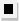Chapter 13.6, Problem 44E### Algebra and Trigonometry (MindTap ...

4th Edition
James Stewart + 2 others
ISBN: 9781305071742

#### Solutions

Chapter
Section### Algebra and Trigonometry (MindTap ...

4th Edition
James Stewart + 2 others
ISBN: 9781305071742
Textbook Problem

# 4 3 - 4 6Factoring Factor using the Binomial Theorem. ( x − 1 ) 5 + 5 ( x − 1 ) 4 + 10 ( x − 1 ) 3 + 10 ( x − 1 ) 2 + 5 ( x − 1 ) + 1

To determine

To find:

Factor (x1)5+5(x1)4+10(x1)3+10(x1)2+5(x1)+1 using Binomial theorem

Explanation

Given:

The expression (x1)5+5(x1)4+10(x1)3+10(x1)2+5(x1)+1

Approach:

Deduce the exponent n of the binomial from number of terms in the given expression. And then compare the given expression to (a+b)n and solve for a,b.

Calculation:

The given expression has 6 terms and we know a binomial with exponent n has n+1 terms in its binomial expansion. Hence the binomial exponent corresponding to given expression is n=5

### Still sussing out bartleby?

Check out a sample textbook solution.

See a sample solution

#### The Solution to Your Study Problems

Bartleby provides explanations to thousands of textbook problems written by our experts, many with advanced degrees!

Get Started